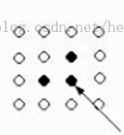# C#图像算法膨胀算法实现## C#代码

```/// <summary>
/// 膨胀算法
/// </summary>
/// <param name="image"></param>
/// <returns></returns>
private Bitmap GetPengZhangImage(Bitmap image)
{
List<Point> setList = new List<Point>();

Bitmap result = image.Clone() as Bitmap;

for (int i = 0; i < result.Width; i++)
{
for (int j = 0; j < result.Height; j++)
{
// 如果应该设置为黑色的
if (SetPixelPengZhang(result, i, j))
{
}
}
}
//膨胀运算
int x, y;
foreach (var item in setList)
{
x = item.X;
y = item.Y;

result.SetPixel(x - 1, y, Color.Black);
result.SetPixel(x, y - 1, Color.Black);
}
return result;
}

// 判断这个点应不应该设置为黑色
protected bool SetPixelPengZhang(Bitmap image, int i, int j)
{
Color c = image.GetPixel(i, j);

if (i != 0 && j != 0)
{
if (image.GetPixel(i, j).ToArgb() == Color.Black.ToArgb())
{
return true;
}
else return false;
}
else return false;
}```

0 打赏

QQ咨询 邮件咨询